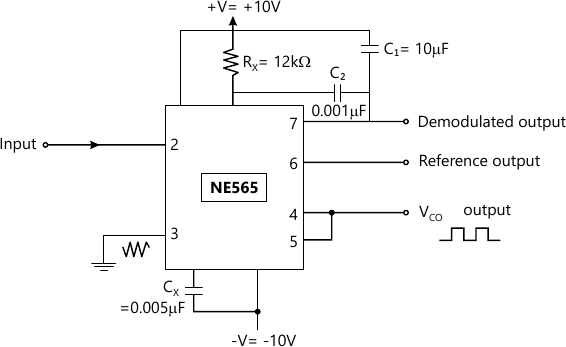MORE IN Integrated Circuits
Total marks: --
Total time: --
INSTRUCTIONS
(1) Assume appropriate data and state your reasons
(2) Marks are given to the right of every question
(3) Draw neat diagrams wherever necessary

Answer any one question from Q1 and Q2
1 (a) Draw the block diagram of op-amp and explain the function of each block in detail
6 M
1 (b) Compare different types of op-amp technologies.
3 M
1 (c) Explain the effect of temperature on:
(i) Input Bias Current
(ii) Input Offset Voltage
(iii) Input Resistance
3 M

2 (a) Find the Q-point VC and IB for dual input balanced output differential amplifier when, RE=RC=65 KΩ. Assume IE=IC, β=100 for both transistors Q1 and Q2; VS=± 12 V.
6 M
2 (b) What is the need of frequency compensation? Explain any one method of frequency compensation.
6 M

Answer any one question from Q3 and Q4
3 (a) Design a lossy integrator with square wave input of 2 Vp-p and 5 Khz frequency. Draw input and output waveforms.
6 M
3 (b) Explain with a neat circuit diagram working of symmetric Schmitt trigger using op-amp. Also derive the equation for the trigger points.
6 M

4 (a) Design an adder using op-amp to get output expression as:
V0= - (2V1 + 3V2+5V3) Where V1, V2 and V3 are inputs.
6 M

Answer any one question from Q5 and Q6
5 (a) With a neat circuit diagram, explain voltage to frequency Converter.
5 M
5 (b) Write a short note on Binary weighted Digital to Analog Converter (DAC).
5 M
5 (c) What output voltage would be produced by a D/A converter whose output range is 0 to 10 V and input binary number is:
(i) 10 (for a 2-bit DAC converter)
(ii) 0110 (for a 4-bit DAC)
(iii) 10111100 (for a 8-bit DAC).
3 M

6 (a) Write a short note on Flash type Analog to Digital Converter.
5 M
6 (b) What are the different types of V to I converter. Explain any one.
5 M
6 (c) List various specification of ADC.
3 M

Answer any one question from Q7 and Q8
7 (a) With the help of neat block diagram explain operation of PLL.
5 M
7 (b) What is the need of current boosting circuit ? Explain with the help of any one circuit.
5 M
7 (c) Draw block diagram of frequency multiplier. Draw input-output waveforms.
3 M

8 (a) For PLL circuit shown in Fig. 1, calculate the following:
(i) Free running frequency
(ii) Lock range
(iii) Capture range6 M
8 (b) Write a short note on practical voltage regulator using LM317.
5 M
8 (c) Explain the following terms: